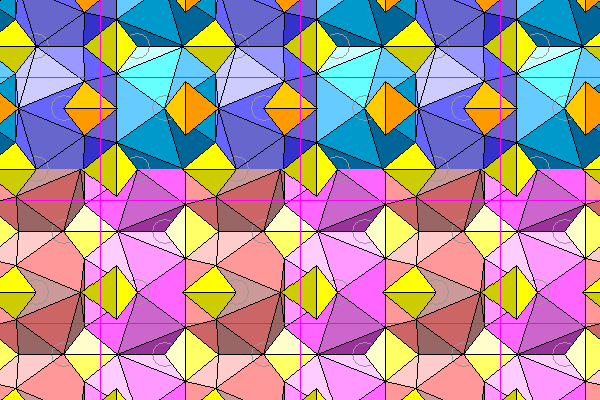# Barite

Steven Dutch, Professor Emeritus, Natural and Applied Sciences, Universityof Wisconsin - Green Bay

With most minerals, coordination polyhedra dramatically improve the visualization of the atomic structure. But in a few cases, coordination polyhedra don't help and may even hinder understanding. Barite (BaSO4) is one such case.

Like many minerals with complex anions, the cations occupy oddly-shaped voids between the anions. The resulting coordination polyhedra are not governed by radius ratios, orbitals, or local symmetry considerations. Instead they are dictated by available space.

The diagram below shows barite, viewed down the crystallographic c axis. The symmetry class is orthorhombic 2/m2/m2/m, but the space group is pbnm, meaning there are glide planes. Thus the yellow and orange sulfate tetrahedra consist of mirror image pairs, but each is offset one half unit cell vertically in the diagram. The green atoms are barium. Lighter colors are farther below the plane of the figure.Here we see several lattice planes viewed slightly obliquely. Again, lighter colors denote increasing distance from the viewer.The view below looks down the a-axis, with b extending horizontally and c extending vertically.The coordination polyhedron of the barium is irregular and hard to visualize. The diagram below shows the oxygen atoms in blue surrounding one barium atom. Lighter colors denote greater distance from the viewer. The barium atom is beneath the central highest oxygen and is slightly offset for visibility. To the right is a coordination polyhedron. Three levels of sulfate groups are shown. The coordination polyhedron shares two oxygens with five sulfate tetrahedra: the four surrounding ones shown plus a fifth at the bottom. Two other tetrahedra share a single atom, bringing the total coordination to 12.The only symmetry the barite coordination polyhedra have is a single mirror plane, visible in the view down the a axis. Thus there are four possible orientations for the polyhedra: two mirror images each reflected in the planes normal to a and b. The four possible polyhedra are shown below. The right polyhedra are the bottoms of the left polyhedra.The view below shows two layers of barium coordination polyhedra. The upper layer is blue, the lower is pink.The actual shape of the barium polyhedron is hard to visualize. Probably the simplest way is to picture it as having a pentagonal base (actually three triangles that are almost coplanar) and a hexagonal cap. The base and cap are joined by a girdle of triangles, except that because of the unequal number of sides, one of the faces is a quadrilateral instead of a triangle.Created 14 April, 2011,  Last Update